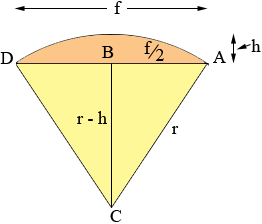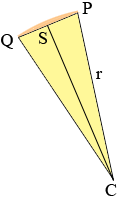SEARCH HOMEMath Central Quandaries & QueriesQuestion from Scott: Given the length of an arc, the rise of an arc, and the number of segments that I need to divide the arc into - how do I determine the length of each segment? Imagine a bay window. It could have 5 side or 7 sides, just as an example. How do I determine the width of each window given that the unit will be mounted into a frame with a 96 inch opening. The rise of the unit will extend out 18 inches. Lets say that the number of single windows unit within the unit is 5. How wide should each window be? Do you understand? Thanks, ScottHi Scott,

I think I understand.

Here is my diagramI am going to develop a general expression showing the quantities you sent in red.

In the diagram f is the width of the opening (f = 96 inches) and h is the rise of the arc (h = 18 inches). C is the centre of the circle that subtends the arc and r is the radius of the circle. Since triangle ABC is a right triangle Pythagoras Theorem gives (f/2)2 + (r - h)2 = r2. Solving for the radius r gives

r = (f2 + 4h2)/(8h) (r = 73 inches).

Again, since triangle ABC is a right triangle sin(angle BCA) = (f/2)/r so (angle BCA) = sin-1(f/(2r) and

angle DCA = 2 sin-1(f/(2r)) (angle DCA = 82.2o)

Now suppose there are to be n window units (n = 5). Each window unit then subtends an angle of measure (angle DCA)/5. Call the measure of this angle to (to = 82.2/5 = 16.4o). In the diagram below the line segment QP represents one window unit and S is the midpoint of QP. Let the width of the window be w units then |PS| = w/2.Since the measure of the angle QCP is to the measure of the angle SCP is to/2. Hence

|PS|/r = w/(2r) = sin(to/2) or

w = 2r sin(to/2) (w = 146 sin(16.4/2) = 20.9 inches).

I hope this helps,
HarleyMath Central is supported by the University of Regina and The Pacific Institute for the Mathematical Sciences.